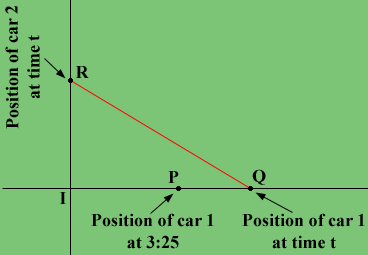SEARCH HOMEMath Central Quandaries & QueriesQuestion from Maryanne, a parent: A car traveling east at 45 mph passes a certain intersection at 3pm. Another car traveling north at 60mph passes the same intersection 25 minutes later. To the nearest minute, figure out when the cars are exactly 40 miles apart.Maryanne,

I am going to let t be time in minutes, starting at 3:25. Car 2 is travelling at 60 mph which is 1 mile per minute and car 1 is travelling at 3/4 miles per minute. My diagram is at some time t minutes after 3:25 when the cars are 40 miles apart.I is the intersection and it took 25 minutes for car 1 to reach P. What is the distance from I to P?

Car 2 travels t minutes to reach R. What is the distance from I to R? What is the distance from P to Q?

Triangle RIQ is a right triangle and the length of QR is 40 miles. What does Pythagoras theorem tell you about the lengths of the sides of the triangle? Solve for t.

I hope this helps,
PennyMath Central is supported by the University of Regina and The Pacific Institute for the Mathematical Sciences.